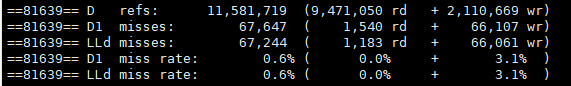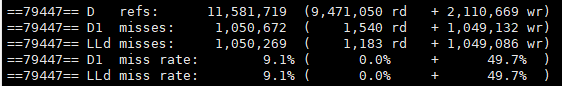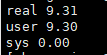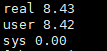## 编译器工程师眼中的好代码（1）：Loop Interchange

2022-08-02Loop Interchange毕昇

## 1. Loop Interchange 相关基本概念 #

### 1.2 Row-major 和 Column-major #

Row-major 和 Column-major 是两种将多维数组存储在线性存储中的方式。数组的元素在内存中是连续的；Row-major ordering 代表行的连续元素在内存中彼此相邻，而 Column-major ordering 则是代表列的连续元素彼此相邻，如下图所示。``````#include <stdio.h>

int main(){
size_t i,j;
const size_t dim = 1024 ;
int matrix [dim][dim];

for (i=0;i< dim; i++)
for (j=0;j <dim;j++)
matrix[i][j]= i*j;

return 0;
}
````````````#include <stdio.h>

int main(){

size_t i,j;

const size_t dim = 1024 ;

int matrix [dim][dim];

for (i=0;i< dim; i++){
for (j=0;j <dim;j++){
matrix[j][i]= i*j;
}
}
return 0;
}
``````### 1.3 Loop Interchange #

Loop Interchange 利用系统倾向于访问内存中较为靠近的值的特征以及 C/C++ Row-major 的特点，通过改变循环嵌套中两个循环之间的执行顺序，增加整体代码空间局部性。此外，它还可以启用其他重要的代码转换，例如，Loop Reordering 就是 Loop Interchange 扩展到两个以上循环被重新排序时的优化。在 LLVM 中，Loop Interchange 需要通过使能`-mllvm -enable-loopinterchange`选项启用。

## 2. 优化示例 #

### 2.1 基础场景 #

``````for(int i = 0; i < 2048; i++) {
for(int j = 0; j < 1024; j++) {
for(int k = 0; k < 1024; k++)  {
C[i * 1024 + j] += A[i * 1024 + k] * B[k * 1024 + j];
}
}
}
``````

``````for(int i = 0; i < 2048; i++) {
for(int k = 0; k < 1024; k++) {
for(int j = 0; j < 1024; j++)  {
C[i * 1024 + j] += A[i * 1024 + k] * B[k * 1024 + j];
}
}
}
``````

``````\$ time -p ./a.out
````````````\$ sudo perf stat -r 3 -e cache-misses,cache-references,L1-dcache-load-misses,L1-dcache-loads ./a.out
``````Loop Interchange 后的结果如下：

``````\$ time -p ./a.out
````````````\$ sudo perf stat -r 3 -e cache-misses,cache-references,L1-dcache-load-misses,L1-dcache-loads ./a.out
``````### 2.2 特殊场景 #

``````for ( int i = 0; i < N; ++ i )
{
if( I[i] != 1 ) continue;

for ( int m = 0; m < M; ++ m )
{
Res2 = res[m][i] * res[m][i];
norm[m] += Res2;
}
}
``````

``````for ( int m = 0; m < M; ++ m )
{
for ( int i = 0; i < N; ++ i)
{

Res2 = res[m][i] * res[m][i];
norm[m] += Res2;

if( I[i] != 1 )  //补充逻辑，保证源代码语义
{
norm[m] -= Res2;
continue;
}
}
}
``````

## 3. 毕昇编译器在 LLVM 社区的贡献 #

``````for (c = 0, e = 1; c + e < 150; c++, e++) {
d = 5;
for (; d; d--)
a |= b[d + e][c + 9];
}
}
``````

``````double matrix[dim][dim];
for (j=0;j< dim; j++)
for (i=0;i <dim;i++)
matrix[i][j] += 1.0;
``````

D120386 [LoopInterchange] Try to achieve the most optimal access pattern after interchange (https://reviews.llvm.org/D120386)

``````void f(int e, int f) {
for (int a = 0; a < 100; a++) {
for (int b = 0; b < 100; b++) {
for (int c = 0; c < 100; c++) {
f[c][b][a] = e[c][b][a];
}
}
}
``````

=>

``````void f(int e, int f) {
for (int c = 0;  c < 100; c++) {
for (int b = 0; b < 100; b++) {
for (int a = 0; a < 100; a++) {
f[c][b][a] = e[c][b][a];
}
}
}
}
``````

D124926 [LoopInterchange] New cost model for loop interchange (https://reviews.llvm.org/D124926)

• D102300 [LoopInterchange] Check lcssa phis in the inner latch in scenarios of multi-level nested loops
• D101305 [LoopInterchange] Fix legality for triangular loops
• D100792 [LoopInterchange] Handle lcssa PHIs with multiple predecessors
• D98263 [LoopInterchange] fix tightlyNested() in LoopInterchange legality
• D98475 [LoopInterchange] Fix transformation bugs in loop interchange
• D102743 [LoopInterchange] Handle movement of reduction phis appropriately during transformation (pr43326 && pr48212)
• D128877 [LoopCacheAnalysis] Fix a type mismatch bug in cost calculation

• D115238 [LoopInterchange] Remove a limitation in legality
• D118102 [LoopInterchange] Detect output dependency of a store instruction with itself
• D123559 [DA] Refactor with a better API
• D122776 [NFC][LoopCacheAnalysis] Add a motivating test case for improved loop cache analysis cost calculation
• D124984 [NFC][LoopCacheAnalysis] Update test cases to make sure the outputs follow the right order
• D124725 [NFC][LoopCacheAnalysis] Use stable_sort() to avoid non-deterministic print output
• D127342 [TargetTransformInfo] Added an option for the cache line size
• D124745 [Delinearization] Refactoring of fixed-size array delinearization
• D122857 [LoopCacheAnalysis] Enable delinearization of fixed sized array

## 参考 #

### 加入我们 #

openEuler 公众号：https://url.cy/01H5Rs

openEuler 官网：https://www.openeuler.org/zh/

【免责声明】本文仅代表作者本人观点，与本网站无关。本网站对文中陈述、观点判断保持中立，不对所包含内容的准确性、可靠性或完整性提供任何明示或暗示的保证。本文仅供读者参考，由此产生的所有法律责任均由读者本人承担。